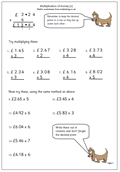# Multiplication of money (1)Here we have a straightforward worksheet dealing with the multiplication of money by a single digit.The main concern here is that the process is carried out much as multiplying a 3 or 4-digit number, but remembering the decimal point.

The second set of questions, from 9. to 15 should be set out in the correct way and not attempted as shown.

It is often a good idea for children to check the answers to these types of page by using a calculator.

Multiplication of money (pg 1)